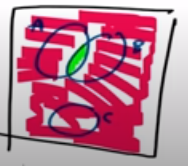Need Help?

Subscribe to Probability

###### \${selected_topic_name}
• Notes

Samples of emissions from three suppliers are classi-
fied for conformance to air-quality specifications. The results
from 100 samples are summarized as follows: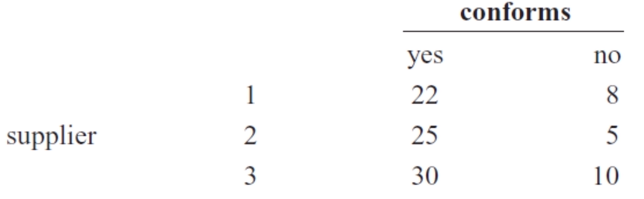Let $A$ denote the event that a sample is from supplier 1 , and let
$B$ denote the event that a sample conforms to specifications.
Determine the number of samples in $A^{\prime} \cap B, B^{\prime},$ and $A \cup B$ .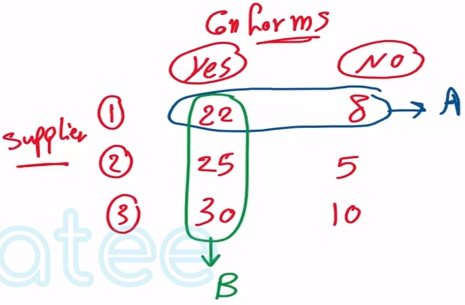$n=100$

$A \rightarrow$ from (1)

B $\rightarrow$ Samples that conferms To specicication

$A^{\prime} \cap B$

$B^{\prime}, A \cup B$

$A^{\prime } \cap B=25+30=55$

$B^{\prime}=8+5+10=23$

$A \cup B=8+22+25+30=85$

Three events are shown on the Venn diagram in the
following figure: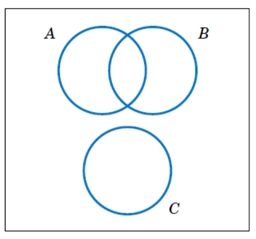Reproduce the figure and shade the region that corresponds to
each of the following events.

(a) $A^{\prime} \quad$ (b) $(A \cap B) \cup\left(A \cap B^{\prime}\right)$
(c) $(A \cap B) \cup C \quad$ (d) $(B \cup C)^{\prime}$
(e) $(A \cap B)^{\prime} \cup C$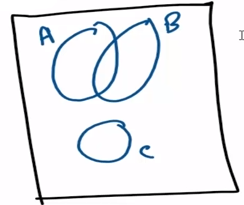a) $A^{\prime}$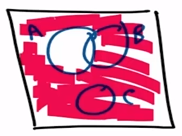b) $(A \cap B) \cup(A \cap B^{\prime})$

A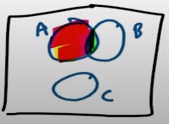c) $(A \cap B) \cup C$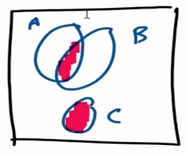d) $(B \cup C)^{\prime}$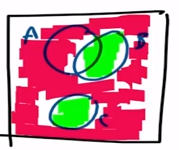e) $(A \cap B)^{\prime} \cup C$